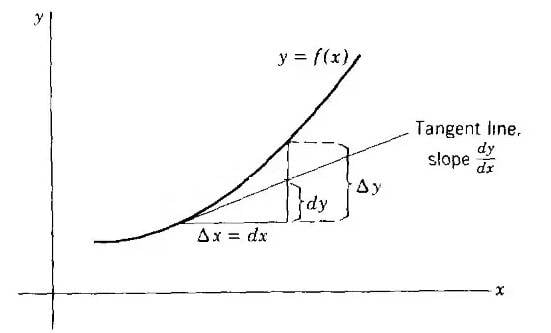# Why is Delta y not equal to dy for infinitesimally dx on the graph?

• B
Mike_bb
TL;DR Summary
Why is Delta y not equal to dy for infinitesimally dx on the graph?
Hello!

As is known, $\Delta y = dy$ for infinitesimally small $dx$. It's true.
But if we have graph we may see that $\Delta y$ isn't equal to $dy$ even for infinitesimally small $dx$. Why is that so?

Thanks!Last edited:

Mentor
2022 Award
Please forget the notation ##dy## and ##dx## at this point in your education. They are no actual distances and are only an abbreviation in this context.

Firstly, they make only sense in the combination ##\dfrac{dy}{dx}## in this case.
Secondly, they only abbreviate a limit: ##\lim_{\Delta x \to 0}\dfrac{\Delta y}{\Delta x}.##

•sysprog
Summary:: Why is Delta y not equal to dy for infinitesimally dx on the graph?

Hello!

As is known, $\Delta y = dy$ for infinitesimally small $dx$. It's true.
But if we have graph we may see that $\Delta y$ isn't equal to $dy$ even for infinitesimally small $dx$. Why is that so?

Thanks!View attachment 301195
Because delta y is the change in y, while dy is only the linear part.

Mike_bb
Because delta y is the change in y, while dy is only the linear part.
Yes. But we can use dy to represent finite Delta y as sum of infinitesimally small dy and it's right.

•PeroK
Mentor
2022 Award
That is exactly why I said: forget about it.

It is a misleading notation until you are willing to learn it rigorously, in which case it becomes complicated. As long as you have to use the term "slope", as long are the actual quantities
$$\Delta x\, , \,\Delta y\, , \,\Delta f(x)\, , \,\dfrac{\Delta y}{\Delta x}\, , \,\lim_{\Delta x \to 0} \dfrac{\Delta y}{ \Delta x}$$
all you actually need. Write it ##y'## and ##f'(x).##

Or learn it correctly. In this case, you should start to read the series I linked to. However, the first part is all you need at this point. Parts 2-5 show you where such a notation leads to.

Homework Helper
Please forget the notation ##dy## and ##dx## at this point in your education. They are no actual distances and are only an abbreviation in this context.

Firstly, they make only sense in the combination ##\dfrac{dy}{dx}## in this case.
Secondly, they only abbreviate a limit: ##\lim_{\Delta x \to 0}\dfrac{\Delta y}{\Delta x}.##
Why would you want to forget about d it is often used.
$$\frac{\mathit{dy}}{\mathit{dx}}=\frac{\mathit{dx}}{\mathit{du}}\frac{\mathit{du}}{\mathit{dx}}$$
$$\int \frac{\mathit{dx}}{a x^2+b x+c}$$
$$u \mathit{dx}=u v-v \mathit{dx}$$
Some people write
$$\int x^2 \phantom{\mathit{dx}}=\frac{1}{3}x^3+C$$
but they know d is there without writing it like
$$x=1x^1$$.
It is not clear that $$y^\prime$$ is a superor notation but regardless one must know all the commonly used notations to avoid confusion.
Would you tell someone to forget $$\sin$$ and write
$$\mathrm{sen}\left(\frac{\pi}{4}\right)=\frac{\sqrt{2}}{2}$$?

Mentor
2022 Award
Why would you want to forget about d it is often used.
Because it confuses you and creates more problems than it solves. The chain rule can easily be phrased with primes instead of differentials, and the expression in an integral is only there to mark the variable anyway.

If you want to use it, then please explain it to me. I know that my definition won't be the one you understand (https://en.wikipedia.org/wiki/One-form; https://www.physicsforums.com/threa...ifferential-forms.1012875/page-2#post-6630386), so give me yours. What are ##df## and ##dx##?

$$\frac{\mathit{dy}}{\mathit{dx}}=\frac{\mathit{dx}}{\mathit{du}}\frac{\mathit{du}}{\mathit{dx}}$$
$$\int \frac{\mathit{dx}}{a x^2+b x+c}$$
$$u \mathit{dx}=u v-v \mathit{dx}$$
Some people write
$$\int x^2 \phantom{\mathit{dx}}=\frac{1}{3}x^3+C$$
but they know d is there without writing it like
$$x=1x^1$$.
It is not clear that $$y^\prime$$ is a superor notation but regardless one must know all the commonly used notations to avoid confusion.
Would you tell someone to forget $$\sin$$ and write
$$\mathrm{sen}\left(\frac{\pi}{4}\right)=\frac{\sqrt{2}}{2}$$?

Last edited:
•robphy